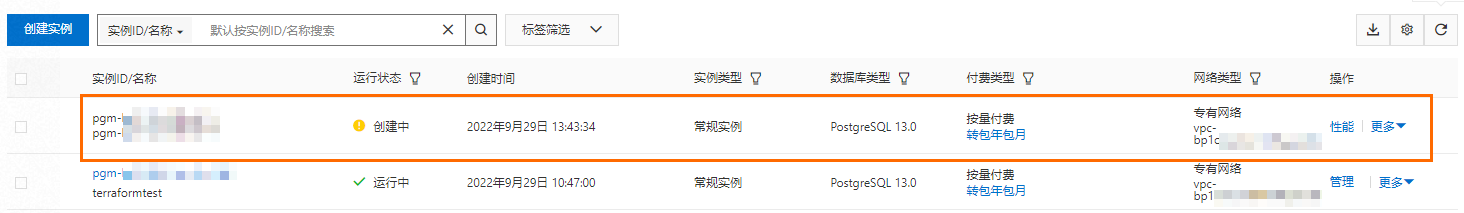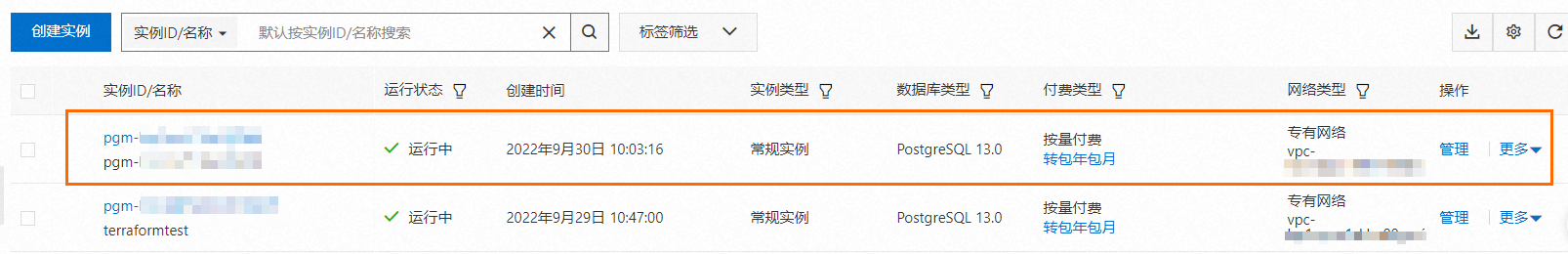## 按时间点恢复实例

1. 在terraform.tf文件中增加`resource "alicloud_rds_clone_db_instance" "example" {}`配置项，具体配置如下：
``````...
resource "alicloud_rds_clone_db_instance" "example" {
source_db_instance_id    = alicloud_db_instance.instance.id
db_instance_storage_type = "cloud_essd"
payment_type             = "PayAsYouGo"
restore_time             = "2022-09-29T04:32:59Z"
db_instance_storage      = "50"
}           ``````
说明 `restore_time`参数配置的取值获取方法：
1. 在terraform.tf文件中增加`data "alicloud_rds_backups" "querybackups" {}`配置项查询备份。
2. 使用terraform apply执行查询。
3. 使用terraform show查询备份信息，获取查询结果中`backup_end_time`的参数取值。

具体操作，请参见查看备份

2. 运行`terraform apply`
出现如下配置信息后，确认配置信息并输入yes，开始恢复实例。
``````alicloud_db_instance.instance: Refreshing state... [id=pgm-****]
alicloud_rds_backup.instance: Refreshing state... [id=pgm-****]
alicloud_db_backup_policy.instance: Refreshing state... [id=pgm-****]
data.alicloud_rds_backups.querybackups: Read complete after 0s [id=128503****]

Terraform used the selected providers to generate the following execution plan. Resource actions are indicated with the following symbols:
+ create

Terraform will perform the following actions:

# alicloud_rds_clone_db_instance.example will be created
+ resource "alicloud_rds_clone_db_instance" "example" {
+ acl                        = (known after apply)
+ auto_upgrade_minor_version = (known after apply)
+ ca_type                    = (known after apply)
+ category                   = (known after apply)
+ connection_string          = (known after apply)
+ db_instance_class          = (known after apply)
+ db_instance_storage        = 50
+ db_instance_storage_type   = "cloud_essd"
+ engine                     = (known after apply)
+ engine_version             = (known after apply)
+ ha_mode                    = (known after apply)
+ id                         = (known after apply)
+ instance_network_type      = (known after apply)
+ maintain_time              = (known after apply)
+ payment_type               = "PayAsYouGo"
+ port                       = (known after apply)
+ private_ip_address         = (known after apply)
+ replication_acl            = (known after apply)
+ restore_time               = "2022-09-29T04:32:59Z"
+ security_ips               = (known after apply)
+ server_cert                = (known after apply)
+ server_key                 = (known after apply)
+ source_db_instance_id      = "pgm-bp1668g9bm4bm9p5"
+ ssl_enabled                = (known after apply)
+ sync_mode                  = (known after apply)
+ tcp_connection_type        = (known after apply)
+ vpc_id                     = (known after apply)
+ vswitch_id                 = (known after apply)
+ zone_id                    = (known after apply)

+ parameters {
+ name  = (known after apply)
+ value = (known after apply)
}
}

Plan: 1 to add, 0 to change, 0 to destroy.

Do you want to perform these actions?
Terraform will perform the actions described above.
Only 'yes' will be accepted to approve.

Enter a value:``````

出现类似如下日志时，表示恢复成功。

``````alicloud_rds_clone_db_instance.example: Creating...
alicloud_rds_clone_db_instance.example: Still creating... [10s elapsed]
...
alicloud_rds_clone_db_instance.example: Still creating... [24m11s elapsed]
alicloud_rds_clone_db_instance.example: Creation complete after 24m34s [id=pgm-****]

Apply complete! Resources: 1 added, 0 changed, 0 destroyed.``````
3. 查看结果。
• 运行`terraform show`查看实例恢复情况。
``````...
# alicloud_rds_clone_db_instance.example:
resource "alicloud_rds_clone_db_instance" "example" {
category                 = "HighAvailability"
connection_string        = "pgm-****.pg.rds.aliyuncs.com"
db_instance_class        = "pg.n2.2c.2m"
db_instance_storage      = 50
db_instance_storage_type = "cloud_essd"
deletion_protection      = false
engine                   = "PostgreSQL"
engine_version           = "13.0"
ha_mode                  = "RPO"
id                       = "pgm-****"
instance_network_type    = "VPC"
maintain_time            = "18:00Z-22:00Z"
payment_type             = "PayAsYouGo"
port                     = "5432"
restore_time             = "2022-09-29T04:32:59Z"
security_ips             = [
"0.0.0.0/0",
]
source_db_instance_id    = "pgm-****"
sync_mode                = "Async"
tcp_connection_type      = "LONG"
vswitch_id               = "vsw-****"
zone_id                  = "cn-hangzhou-h"
}``````
• 登录RDS控制台查看实例恢复情况。## 按备份集恢复实例

1. 在terraform.tf文件中增加`resource "alicloud_rds_clone_db_instance" "example" {}`配置项，具体配置如下：
``````...
resource "alicloud_rds_clone_db_instance" "example" {
source_db_instance_id    = alicloud_db_instance.instance.id
db_instance_storage_type = "cloud_essd"
payment_type             = "PayAsYouGo"
backup_id                = "1499356764"
db_instance_storage      = "50"
}           ``````
说明 `backup_id`参数配置的取值获取方法：
• 在terraform.tf文件中增加`data "alicloud_rds_backups" "querybackups" {}`配置项查询备份。
• 使用terraform apply执行查询。
• 使用terraform show查询备份信息，获取查询结果中`backup_id`的参数取值。

具体操作，请参见查看备份

2. 运行`terraform apply`
出现如下配置信息后，确认配置信息并输入yes，开始恢复实例。
``````alicloud_db_instance.instance: Refreshing state... [id=pgm-****]
alicloud_db_backup_policy.instance: Refreshing state... [id=pgm-****]
data.alicloud_rds_backups.querybackups: Read complete after 0s [id=5945****]

Terraform used the selected providers to generate the following execution plan. Resource actions are indicated with the
following symbols:
+ create

Terraform will perform the following actions:

# alicloud_rds_clone_db_instance.example will be created
+ resource "alicloud_rds_clone_db_instance" "example" {
+ acl                        = (known after apply)
+ auto_upgrade_minor_version = (known after apply)
+ backup_id                  = "1499356764"
+ ca_type                    = (known after apply)
+ category                   = (known after apply)
+ connection_string          = (known after apply)
+ db_instance_class          = (known after apply)
+ db_instance_storage        = 50
+ db_instance_storage_type   = "cloud_essd"
+ engine                     = (known after apply)
+ engine_version             = (known after apply)
+ ha_mode                    = (known after apply)
+ id                         = (known after apply)
+ instance_network_type      = (known after apply)
+ maintain_time              = (known after apply)
+ payment_type               = "PayAsYouGo"
+ port                       = (known after apply)
+ private_ip_address         = (known after apply)
+ replication_acl            = (known after apply)
+ security_ips               = (known after apply)
+ server_cert                = (known after apply)
+ server_key                 = (known after apply)
+ source_db_instance_id      = "pgm-****"
+ ssl_enabled                = (known after apply)
+ sync_mode                  = (known after apply)
+ tcp_connection_type        = (known after apply)
+ vpc_id                     = (known after apply)
+ vswitch_id                 = (known after apply)
+ zone_id                    = (known after apply)

+ parameters {
+ name  = (known after apply)
+ value = (known after apply)
}
}

Plan: 1 to add, 0 to change, 0 to destroy.

Do you want to perform these actions?
Terraform will perform the actions described above.
Only 'yes' will be accepted to approve.

Enter a value:``````

出现类似如下日志时，表示恢复成功。

``````alicloud_rds_clone_db_instance.example: Creating...
alicloud_rds_clone_db_instance.example: Still creating... [10s elapsed]
...
alicloud_rds_clone_db_instance.example: Still creating... [19m31s elapsed]
alicloud_rds_clone_db_instance.example: Creation complete after 19m39s [id=pgm-bp1sox718o31j940]

Apply complete! Resources: 1 added, 0 changed, 0 destroyed.``````
3. 查看结果。
• 运行`terraform show`查看实例恢复情况。
``````...
# alicloud_rds_clone_db_instance.example:
resource "alicloud_rds_clone_db_instance" "example" {
backup_id                = "1499356764"
category                 = "HighAvailability"
connection_string        = "pgm-****.pg.rds.aliyuncs.com"
db_instance_class        = "pg.n2.2c.2m"
db_instance_storage      = 50
db_instance_storage_type = "cloud_essd"
deletion_protection      = false
engine                   = "PostgreSQL"
engine_version           = "13.0"
ha_mode                  = "RPO"
id                       = "pgm-****"
instance_network_type    = "VPC"
maintain_time            = "18:00Z-22:00Z"
payment_type             = "PayAsYouGo"
port                     = "5432"
• 登录RDS控制台查看实例恢复情况。